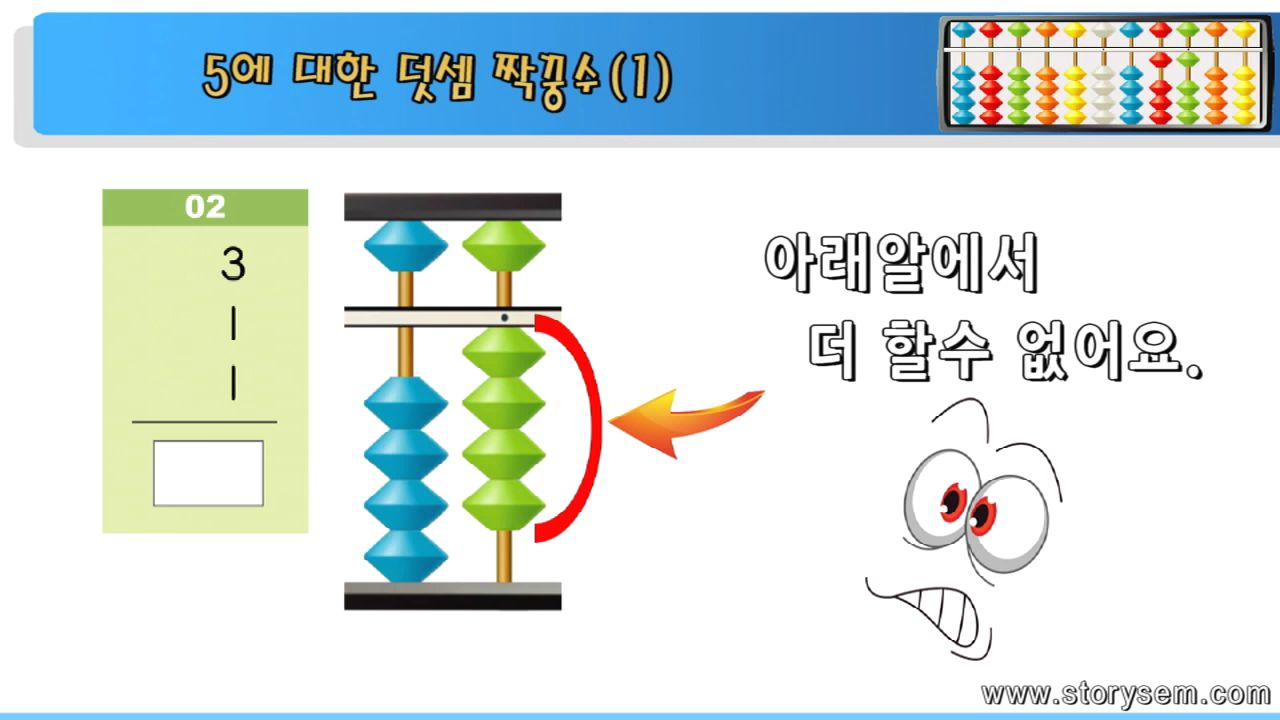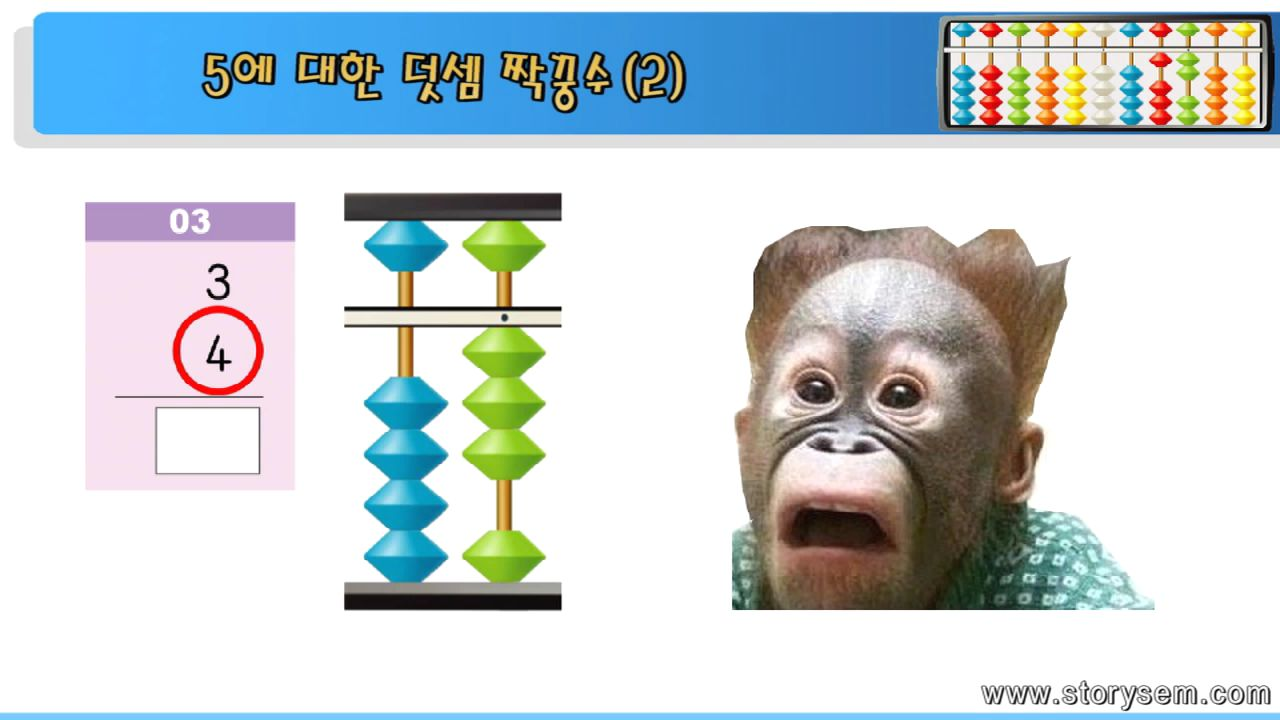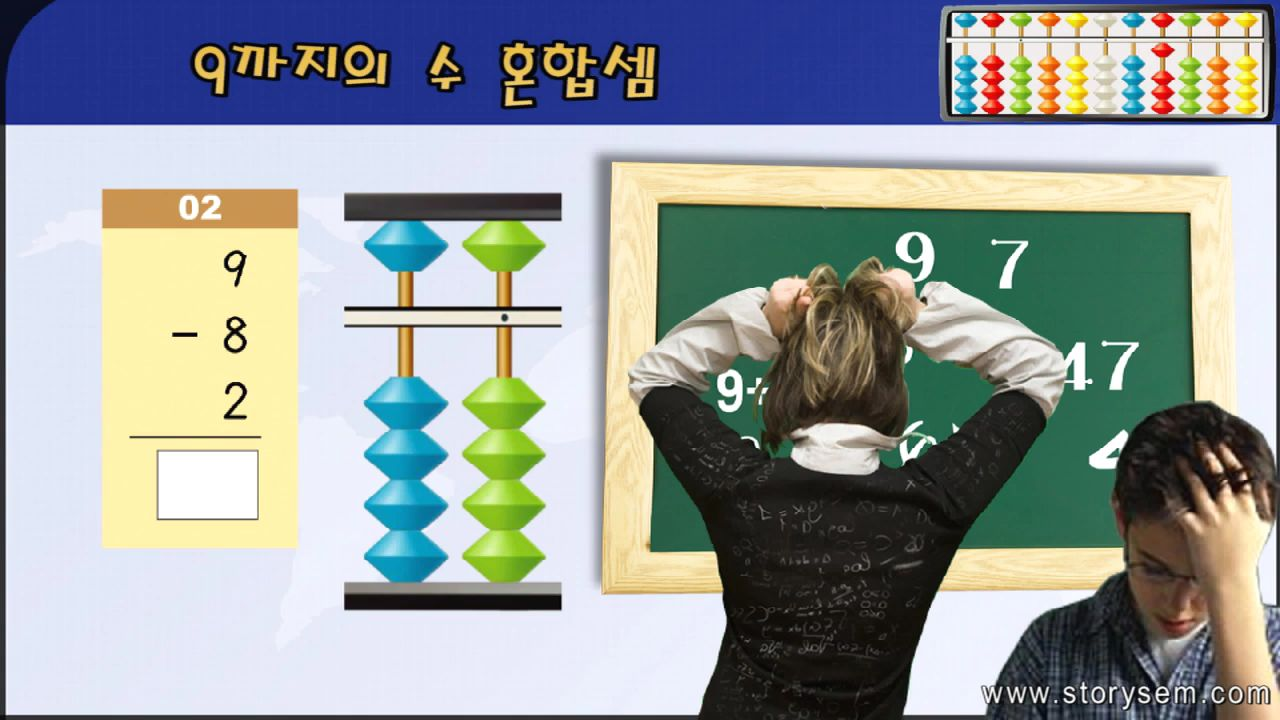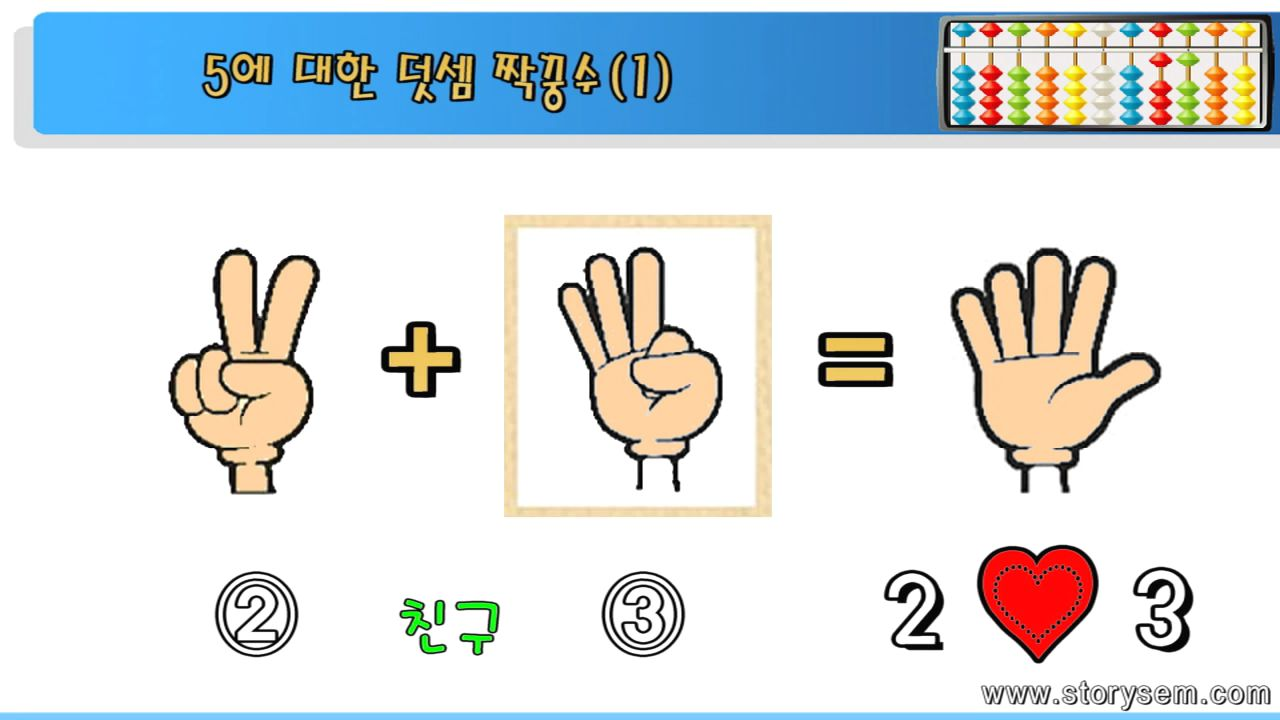#### 프로그램

주산으로 시작하는 수학영재 만들기 프로그램!
프로그램 소개Can learn abacus not boring with multimedia, graphics, subtitles, the motion of the character.Can calculate arithmetic operation easily and fun through the decomposition and combination of number.Adds a number and factor of an abacus to the animation of friends anthropomorphic abacus bead, stimulates curiosity and interest and approaches to learning with fun without resistance.Adds interest in math with animated storytelling.In parables and examples related to the number, it improves greatly mental arithmetic skills, calculation ability, logicality and thinking skills.The best means for learning the scientific understanding of mathematical concepts can be utilized for the abacus.
학습절차목표 달성 진도Can learn abacus not boring with multimedia, graphics, subtitles, the motion of the character.Can calculate arithmetic operation easily and fun through the decomposition and combination of number.Adds a number and factor of an abacus to the animation of friends anthropomorphic abacus bead, stimulates curiosity and interest and approaches to learning with fun without resistance.Adds interest in math with animated storytelling.In parables and examples related to the number, it improves greatly mental arithmetic skills, calculation ability, logicality and thinking skills.The best means for learning the scientific understanding of mathematical concepts can be utilized for the abacus.